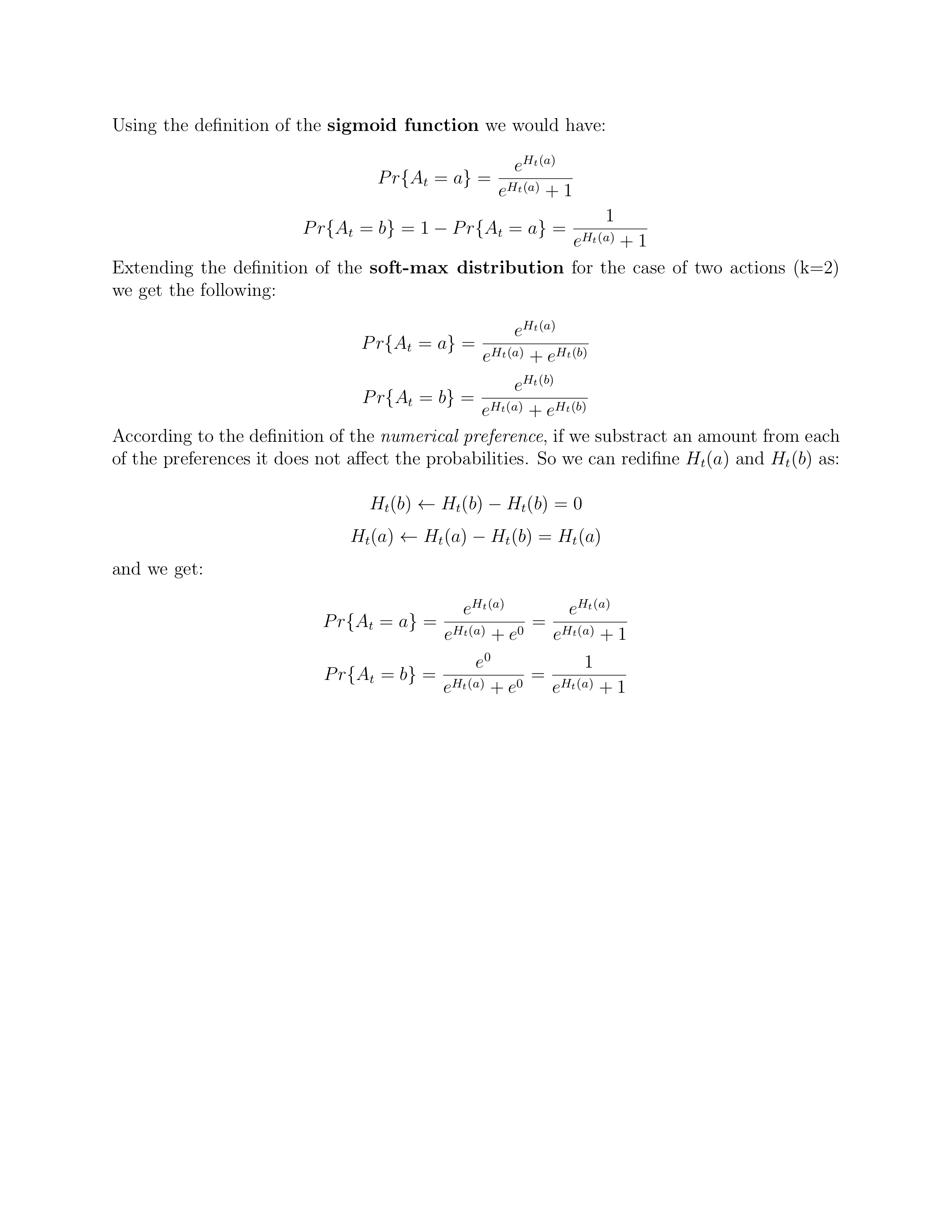## 前言

https://github.com/iamhectorotero/rlai-exercises

https://github.com/LyWangPX/Reinforcement-Learning-2nd-Edition-by-Sutton-Exercise-Solutions

https://rl.qiwihui.com/zh_CN/latest/index.html

## 第二章

### 练习2.1

Q：在$\epsilon$贪心动作选择中，在有两个动作及$\epsilon=0.5$的情况下，贪心动作被选择的概率是多少？

A：0.5的概率开发，然后选择贪心动作。0.5的概率试探，试探时有0.5概率选择贪心动作。$0.5+0.5*0.5=0.75$

### 练习2.2

Q：赌博机的例子 考虑一个$k=4$的多臂赌博机问题，记做1，2，3，4。 将一个赌博机算法应用与这个问题，算法使用$\epsilon$贪心动作选择，基于采样平均的动作价值估计，对于所有a，初始估计为 Q1(a)=0。假设动作及收益的最初顺序是 A1=1，R1=1，A2=2， R2=1，A3=2，R3=2，A4=2， R4=2，A5=3，R5=0。在其中的某些案例中可能发生了$\epsilon$的情形导致一个动作被随机选择。请回答，在哪些时刻中这种情形肯定发生了？在哪些时刻中这些情形可能发生了？

A：45肯定发生了，123可能发生。

### 练习2.3

Q：在图2.2所示的比较中，从累积收益和选择最佳动作的可能性的角度考虑，哪种方法会在长期表现最好？好多少？定量地表达你的答案。

A：Epsilon-0.01长期表现更好，0.01有99%的概率选择最优动作，而0.1只有90%概率选择最优动作

### 练习2.4

Q：如果步长参数$\alpha_n$不是常数，那么估计的$Q_n$就是对于之前得到的收益的加权平均，其权值与公式（2.6）给出的不同。作为对公式2.6的推广，一般情况下，对于这样的步长参数序列，之前的每一次收益的部长分别是多少？

A：1/n，见公式2.3

### 练习2.6

Q：神秘的尖峰 图2.3中展示的结果应该是相当可靠的，因为他们是2000个独立随机的10臂赌博机任务的平均值。那么为什么乐观初始化方法在曲线的早期会出现振荡和峰值呢？换句话说，是什么使得这种方法在特定的早期步骤中表现的特别好或更糟？

A：10臂，对于最好的那个，价值估计下降比较慢，当其他9个下降很快低于最优的那个估计时，会一直选择最优动作。当最优动作下降时，其他9个需要不停的采样下降，9个都下降到比最优还低需要一定的时间，在这段时间里，最优的臂不会被选择。前面的两种过程反复交替，出现震荡。

### 练习2.7

Q：无偏恒定步长 在本章的大多数案例中，我们使用采样平均来估计动作的价值，这是因为采样平均不会像恒定步长那样产生偏差，参见式2.6中的分析。然而，采样平均并不是完全令人满意的解决方案。在非平稳的问题中，他可能会表现的很差。我们是否有办法技能既能利用恒定步长在非平稳过程中的优势，又能有效避免他的误差呢？一种可行的办法是利用如下的步长来处理某个特定动作的第n个收益

$$\beta_n = \frac{\alpha}{\overline o_n}$$

$$\overline o_n = \overline o_{n-1} + \alpha\left ( 1- \overline o_{n-1} \right )$$

A：

$$\overline o_{n}-1=\left( 1-\alpha \right) \left( \overline o_{n-1}-1\right)$$

$$\overline o_{n}=1-\left( 1-\alpha \right) ^{n}$$

$$\begin {split} Q_{n+1} &= Q_{n}+\dfrac {\alpha }{\overline o_n}\left[ R_{n}-Q_{n}\right] \\ &= \dfrac {\alpha }{\overline o_n}R_{n}+\left( 1-\dfrac {\alpha }{\overline o_n}\right) Q_{n}\\ &= \dfrac {\alpha }{\overline o_n}R_{n} + \frac{\left ( 1-\alpha\right ) \overline o_{n-1}}{\overline o_n} \begin{bmatrix}\frac{\alpha}{\overline o_{n-1}}+\left ( 1-\frac{\alpha}{\overline o_{n-1}}\right )Q_{n-1}\end{bmatrix}\\ &= \dfrac {\alpha }{\overline o_n}R_{n}+\dfrac {\alpha \left ( 1-\alpha\right )}{\overline o_n}R_{n-1}+ \begin{bmatrix}\frac{\left ( 1-\alpha\right )^2 {\overline o_{n-2}}}{\overline o_n}\end{bmatrix}Q_{n-1} \\ &=\sum_{i=1}^{n} {\frac{\alpha \left ( 1-\alpha\right )^{n-i}}{\overline o_n}R_i} + \frac{\left ( 1-\alpha\right )^n \overline o_0}{\overline o_n}Q_1 \end {split}$$

### 练习2.8

Q：USB尖峰 在图2.4中，UCB算法的表现在第11步的时候有一个非常明显的尖峰。为什么会产生这个尖峰呢？请注意，你必须同时解释为什么收益在第11步时会增加，以及为什么在后续的若干步中会减少，你的答案才是令人满意的。提示如果$c=1$，那么这个尖峰就不会那么突出了。

A：前10次把每个臂都试了一次，第11次时很有可能选择最优臂，出现峰值。第11次被选中的臂右边值变小，导致难以被再次选中，后面几次选择较差臂，平均收益开始下降。当c=1时，探索的影响因素小了，导致好的臂在后续几次很有可能再次被选中，使尖峰不突出

### 练习2.9

Q：证明在两种动作的情况下，softmax分布与通常在统计学和人工神经网络中使用的logistic或sigmoid函数给出的结果相同

A：### 练习2.10

Q：Suppose you face a 2-armed bandit task whose true action values change randomly from time step to time step. Specifically, suppose that, for any time step, the true values of action 1 and 2 are respectively 0.1 and 0.2 with probability 0.5 (case A), and 0.9 and 0.8 with probability 0.5 (case B). If you are not able to tell which case you face at any step, what is the best expectation of success you can achieve and how should you behave to achieve it? Now suppose that on each step you are told whether you are facing case A or case B (although you still don’t know the true action values). This is an associative search task. What is the best expectation of success you can achieve in this task, and how should you behave to achieve it?

A：第一种情况下，无法确定处于情况A还是B，此时不管选哪个臂，期望成功值都是$0.5 = 0.50.1+0.50.9=0.50.2+0.50.8$，只需随机选择臂即可。